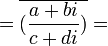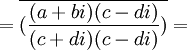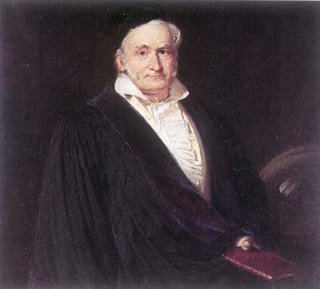## Friday, June 10, 2005

### Norms for Gaussian Integers

In my last blog, I spoke about the importance of unique factorization of integers. This is the idea that all integers can be broken up into a unique set of prime numbers. In today's blog, I will go over some basic lemmas regarding norms. I introduced norms and conjugates in a previous blog.

Carl Friedrich Gauss and Leonhard Euler both proposed extensions of integers that used irrational numbers that they were able to use to make elegant proofs for Fermat's Last Theorem.

A set of integers is extended by including additional values. In standard integers, the values are positive and negative whole numbers and 0. In extended integers, we can include additional values such as i, 5i, etc. Gaussian integers include all of the standard integers plus the addition of multiples of i. In other words, a Gaussian Integer is defined by two integers: a,b where each Gaussian integer can be represented a + bi.

In my previous blog, I spoke about using a special function, the norm, to reason about Gaussian Integers. I will now present a few interesting lemma. To distinguish between Gaussian Integers and standard integers, I will use Greek letters to represent Gaussian Integers and Latin letters to represent standard integers:

Lemma 1: Norm(α) = 0 if and only if α = 0

(1) Norm(α) = Norm(a + bi) = (a + bi)(a - bi) = a2 + b2
(2) But if a ≠ 0, then a2 + b2 > 0.
(3) And the same holds true if b ≠ 0.

QED

In mathematical notation, the conjugate of a Gaussian Integer is represented with an overline over it. So, if α is a Gaussian Integer, then: α is the conjugate. This is important since the norm is equal to a Gaussian Integer multiplied by its conjugate.

Lemma 2: (α * β) = α * β

(1) (α * β) = (a + bi) * (c + di) = (ac - bd) + i(ad + bc)
(2) So, α * β = (ac - bd) - i(ad + bc)
(3) And, α * β = (a - bi) * (c - di) = (ac - bd) - i(ad + bc).
QED

Lemma 3: Norm(α*β) = Norm(α) * Norm(β)

(1) Norm(α*β) = (α*β)(α*β) =
(2) = α*β*α*β [ From Lemma 2 ]
(3) = (α*α)(β*β)
(4) =Norm(α)*Norm(β)

QED

In standard integers, we speak about 1, -1 as being units -- that is, numbers which divide all other numbers. This is important because when we are discussing prime numbers or relatively prime numbers, we are necessarily talking about numbers other than 0 or the units.

In the realm of Gaussian Integers, a unit is defined as any number that divides 1.

Lemma 4: if α is a unit, then Norm(α) = ±1

Proof:

(1) If α is a unit, then there exists another value β such that α * β = 1.
(2) Now, Norm(α * β) = Norm(1) = 1 = Norm(α) * Norm(β)
(3) But if either Norm(α) ≠ ±1 or Norm(β) ≠ ± 1, then the Norm(α * β) couldn't equal 1 (since there are no other rational integers that multiply to 1).
(4) So, Norm(α) must equal ± 1.

QED

Corollary 4.1: The only units for Gaussian Integers are i,-i,1,-1

Proof:

(1) N(i) = i*(-i) = 1 [See Definition 2 here for details if needed]

(2) N(-i) = (-i)*i = 1 [See Definition 2 here for details if needed]

(3) N(1) = 1*1 = 1 [See Definition 2 here for details if needed]

(4) N(-1) = (-1)*(-1) = 1 [See Definition 2 here for details if needed]

(5) If N(α) is a unit where α = a + bi, then:]

(a + bi)(a - bi) = a2 + b2 = 1

(6) But this can only occur if a=0 or b=0 since:

(a) if a ≠ 0 and b≠ 0

(b) Then abs(a) ≥ 1 and abs(b) ≥ 1

(c) And, a2 + b2 ≥ 2

(7) But then the only possible units are 1, -1, i, -i

(a) if a = 0, then b = ± 1 so that we have α = i or α = -i

(b) if b=0, then a = ± 1 so that we have α=1 or α=-1

QED

We are now ready to define a Gaussian Prime. A Gaussian prime is any Gaussian Integer which is not 0 or a unit which is only divisible by a itself or a unit.

Lemma 5: if Norm(α) is a standard prime, then α is a prime.

(1) So, we start with Norm(α) = p which is a standard prime.
(2) Assume α is not a prime.
(3) Then there exists two Gaussian Integers: β, γ that are not units such that: α = β * γ.
(4) And then Norm(β * γ) = Norm(β) * Norm(γ) [By Lemma 3 above]
(5) Since Norm is a mapping function to standard integers, we know that there exists b,g such that:
b = Norm(β)
g = Norm(γ)

(6) We know that Norm(β * γ) = p and we know that b,q ≠ 1 [By Lemma 4, since they are not units]
(7) But then p = b * g which is impossible since p is a prime.
(8) Therefore, we reject our assumption in (2).

QED

Lemma 6: (α/β) = α / β

(1) There exists a,b,c,d such that α = a + bi. β = c + di.

(2) (α/β) =[From Lemma 2 above]= (a + bi) / (c + di)

QED

Lemma 7: Norm(α / β) = Norm(α) / Norm(β)

(1) Norm(α / β) = (α / β) * (α / β) [Definition of Norm]

= (α / β) * α / β = Norm(α) / Norm (β)

QED

Corollary 7.1: If α divides β, then Norm(α) divides Norm(β)

Proof:

(1) Assume that α divides β.

(2) Then there exists an integer γ such that γ = β / α.

(3) By the definition of a norm (see here), Norm(γ) is a rational integer.

(4) But then Norm(β)/Norm(α) = Norm(γ) which means that Norm(α) must divide Norm(β).

QED

In my next blog, I will prove that Gaussian Integers possess unique factorization.

The lemmas from today's blog were taken from an excellent book by Harold M. Stark called An Introduction to Number Theory .

## Thursday, June 09, 2005

### Unique Factorization

Carl Friedrich Gauss was the first mathematician to understand the importance of unique factorization in relation to algebraic integers. While unique factorization was a known property of integers since the time of Euclid, it is not true that it is necessarily a property of all algebraic integers.

Unique factorization is the idea that a given whole number consists of a unique set of primes. For every set of unique primes, there is one and only one number that can be built. For every number, there is one and only one unique set of primes.

Before Gauss, this idea was probably not interesting to mathematicians because it is so clearly true in the case of integers. On the other hand, when Leonhard Euler presented his proof for Fermat's Last Theorem n=3, he introduced a new set of integers in the form of a + b√-3. This was a brilliant proof and yet he made a mistake. He assumed that unique factorization automatically applied. It was Gauss's insight that this assumption requires proof.

Gauss took the idea of unique factorization very seriously when he proposed what are today called Gaussian Integers. Using i-notation, where i is the square root of -1, a Gaussian integer is defined by the set of all values that can be created by a + bi where a,b are integers. It is also represented by the form Z[i]. Using this notation, Euler's proof used integers of the form Z[√-3].

The interesting idea behind Gaussian Integers is that any theorem which is true of all Gaussian Integers is also true of all integers since integers are Gaussian Integers wheren b = 0.

But how does one reason with Gaussian Integers? On the surface, it doesn't look like they will be offer any insights beyond the standard integers. In fact, it seems, on the surface, that reasoning about them will be more difficult since they themselves are more complicated. Using standard integers, we know that 5 is a prime. But in the realm of Gaussian Integers, it is not a prime since
5 = (2 + i)(2 - i) = 4 + 1 = 5.

Gaussian Integers are interesting because they allow us to factor equations in interesting ways. For example, the equation x4 + y4 can now be factored into (x2 - y2i)(x2 + y2i). Without Gaussian Integers, there wouldn't be an easy factoring. This was really Euler's motivation for extending the integers since he was able to break x3 + y3 into a form such as:
(x + y)(x + ay)(x + by).

Coming up with proofs about Gaussian integers becomes interesting with the introduction of a special function called the norm. The norm is a mapping function that maps any Gaussian Integer to a standard integer. The norm is important because it enables to us to define Gaussian primes and because it then enables us to establish unique factorization for Gaussian Integers.

How does one find a mapping function that is guaranteed to convert any Gaussian Integer to a standard integer? The answer comes from the following mathematical equation:

(a + b)(a - b) = aa -ab +ab - bb = a2 - b2

In the case of i, this becomes:

(a + bi)(a - bi) = a2 - (b2)(-1) = a2 + b2.

The form a - bi is called the conjugate of the Gaussian Integer. Hardy and Wright (see reference below) define the norm as the product of a Gaussian Integer with its conjugate. For purposes of my discussion on Gaussian integers, I will use the Hardy and Wright definition (I should point out that this is not the standard definition of norm, see here; I will revisit this point at a later time). The important point behind the norm is that it is a mapping between the Gaussian integers and the rational integers that allows us to reason about the Gaussian integers (see here for an example of using the norm for Gaussian integers to establish a division algorithm for Gaussian integers.)

For example, the norm of 5i is (0 + 5i)(0 - 5i) = 25. Interestingly, the norm of -5i is also 25. This is important because it shows that the norm is not a one-to-one mapping. All Gaussian integers and their conjugates share the same norm.

In summary, I offer the following definitions for Gaussian Integers:

Definition 1: Conjugate of a Gaussian Integer

The conjugate of a Gaussian Integer a+bi is a-bi and likewise, the conjugate of a Gaussian Integer a-bi is a+bi.

Definition 2: Norm of a Gaussian Integer: N(α) = α * α

Following Hardy and Write (see article by Eric Weisstein for details), the norm of a Gaussian integer α is α multiplied by its conjugate α, that is, the norm N(α) = α * α.

Over the next set of blogs, I will show how norms allow us to establish unique factorization for Gaussian Integers. My next blog will cover some basic ideas about Gaussian Integer norms.

References

## Tuesday, June 07, 2005

### Carl Friedrich GaussCarl Friedrich Gauss was born on April 17, 1777 to poor, working class parents in Brauschweig, Germany. His mother had been a maid and his father had been a laborer and handyman. His father, it is said, did not appreciate Gauss's abilities or his later accomplishments.

Gauss is considered to be one of the three greatest mathematicians of all time. The other two being Archimedes and Sir Isaac Newton. His accomplishments are so vast that this blog will only be a brief glimpse. For those interested in greater details, please check out the reference list at the end of this blog.

Gauss showed indications of mathematical genius very early. According to a popular story, he noticed a mistake in his father's calculations when he was only 3 years old. Later, he supposedly taught himself to read and by the time he was 8, he was already astounding people by his mathematical abilities. For example, there is a well known anecdote where a teacher gave his students an assignment to add up a series of 100 numbers. Instantly, Gauss said that he had completed the exercise (the story goes that he had figured that 100 numbers could be determined by the equation n(a+b)(1/2)=50(a+b) where n=100, a = the first digit in the sequence and b = the last digit in the sequence.)

Gauss's teachers would lend him books and try to help him along with his independent studies of the subject. In 1788, at the age of 11, he entered the Gymnasium, a college preparatory school. In 1792, at the age of 14, he received a stipend from the Duke of Brunswick which made him financially independent for the next 16 years and it was that year that he entered Brunswick College.

He entered the University at Gottigen in 1795 and became famous in 1796 at the age of 19 with his discovery on March 30 of a method for constructing a heptadecagon (a regular 17-sided polygon) using only a compass and ruler. He was so excited by this that he requested that a heptadecagon be placed on his tombstone. For his doctoral thesis, he proved the fundamental theorem of algebra which states that all polynomials of degree n have n different solutions in the domain of complex numbers.

Gauss's other discoveries include the law of quadratic reciprocity, Gaussian distribution, modular arithmetic, non-Euclidean geometry, the prime number theorem, the systematization of number theory, and the method of least squares. Gauss worked on many of these discoveries completely on his own without any collaborators.

Gauss also had a strong interest in astronomy. In 1801, the planetoid Ceres was first sighted. Gauss studied data abouts its orbit and was able to predict a second possible sighting which was confirmed on December 31, 1801. In 1805, he became Director of the Observatory in Gottingen.

Despite his fame in mathematics, Gauss never became a mathematics professor and only attended one math conference. It was said that he strongly disliked teaching. When his good friend Farkas Bolyai told him that his son has come up with a non-Euclidean geometry, Gauss responded:
"To praise it would amount to praising myself. For the entire content of the work ... coincides almost exactly with my own meditations which have occupied my mind for the past thirty or thiry-five years."

Gauss's personal life was filled with tragedy. His first wife died in 1809 and a few years later, his first son died. It was said that throughout his life, he never overcame the sadness of this event. His second wife died in 1831 after a long illness. He never married after that. He died on February 23, 1855.

Gauss did not publish most of his discoveries. They were published after his death.

His face can be found on the German Deutche mark from 1989 until 2001:References for this blog: